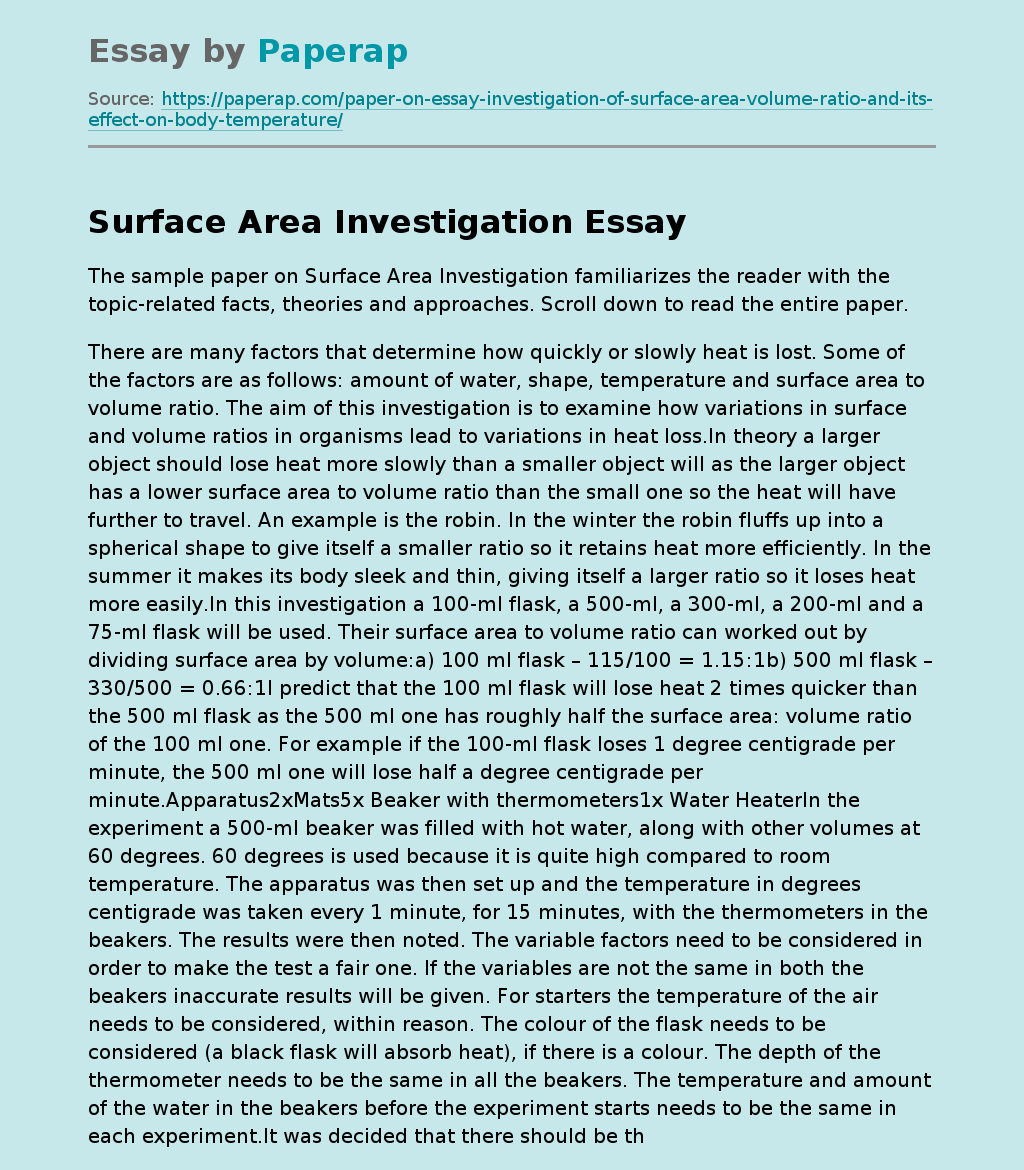# Surface Area Investigation

The sample paper on Surface Area Investigation familiarizes the reader with the topic-related facts, theories and approaches. Scroll down to read the entire paper.

There are many factors that determine how quickly or slowly heat is lost. Some of the factors are as follows: amount of water, shape, temperature and surface area to volume ratio. The aim of this investigation is to examine how variations in surface and volume ratios in organisms lead to variations in heat loss.In theory a larger object should lose heat more slowly than a smaller object will as the larger object has a lower surface area to volume ratio than the small one so the heat will have further to travel.

An example is the robin. In the winter the robin fluffs up into a spherical shape to give itself a smaller ratio so it retains heat more efficiently. In the summer it makes its body sleek and thin, giving itself a larger ratio so it loses heat more easily.

In this investigation a 100-ml flask, a 500-ml, a 300-ml, a 200-ml and a 75-ml flask will be used. Their surface area to volume ratio can worked out by dividing surface area by volume:a) 100 ml flask – 115/100 = 1.15:1b) 500 ml flask – 330/500 = 0.66:1I predict that the 100 ml flask will lose heat 2 times quicker than the 500 ml flask as the 500 ml one has roughly half the surface area: volume ratio of the 100 ml one. For example if the 100-ml flask loses 1 degree centigrade per minute, the 500 ml one will lose half a degree centigrade per minute.

Get quality help nowDoctor JenniferVerified

Proficient in: Experiment5 (893)

“ Thank you so much for accepting my assignment the night before it was due. I look forward to working with you moving forward ”+84 relevant experts are online